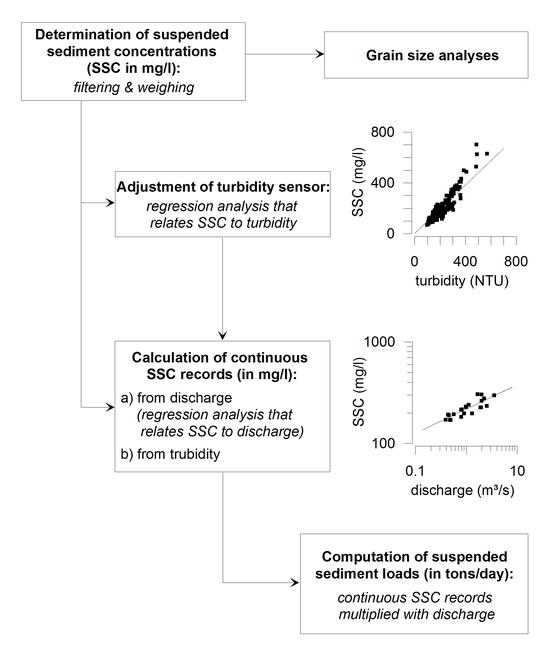Figure 3View Original Image at Full Size

Figure 3. Flowchart of the workflow to calculate sediment loads from measurements of discharge, suspended sediment concentration and turbidity. After suspended sediment concentrations (SSC) and grain size distributions had been determined, continuous SSC records were calculated from turbidity and discharge measurements. Sediment loads were then computed by multiplying continuous SSC records with discharge.

Image 36314 is a 2265 by 1902 pixel JPEG